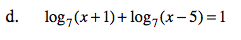Home > CCA2 > Chapter 10 > Lesson 10.2.1 > Problem10-103

10-103.
1. Use the properties of logarithms to rewrite each equation and then solve. Check for extraneous roots. Homework Help ✎

1. log(x) + log(x + 21) = 2

2. 2log4(x) − log4(3) = 2

3. log2(9x + 5) − log2(x2 − 1) = 2

4. log7(x + 1) + log7(x − 5) = 1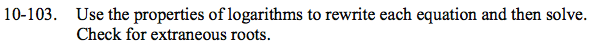See the homework help for problem 10-95.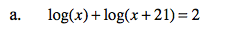x = 4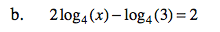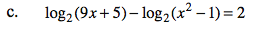x = 3